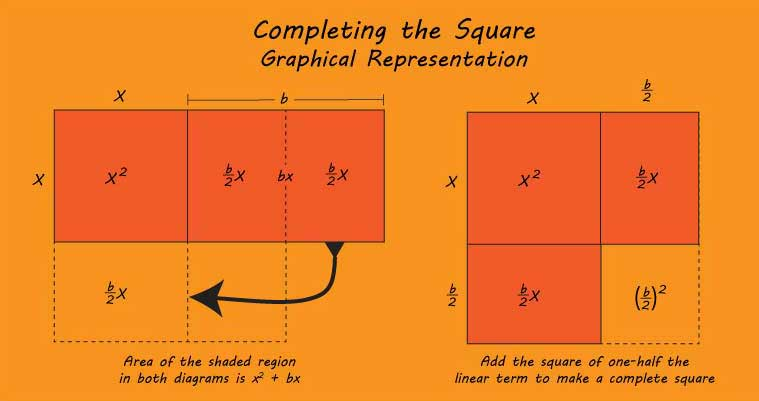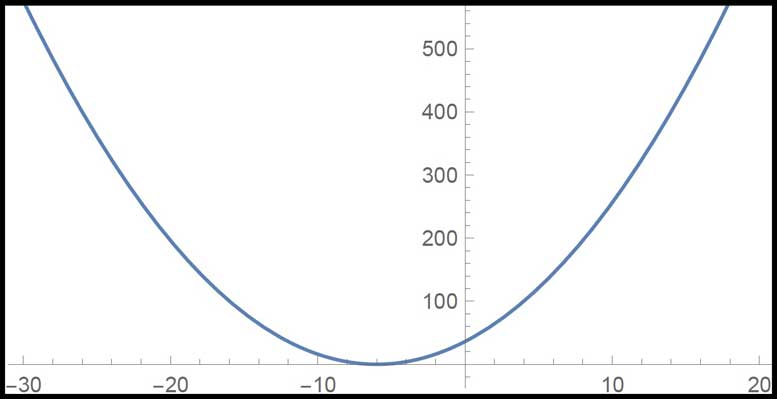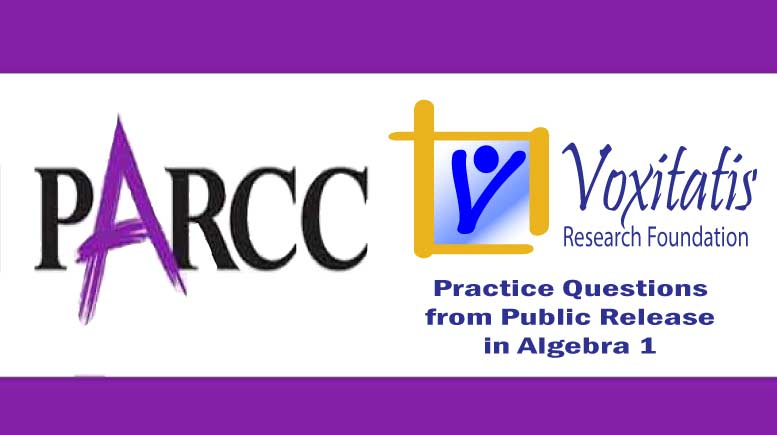Wednesday, November 25, 2020# Algebra 1 PARCC question: complete the square

-

#### The following multi-part constructed response question, explained here in hopes of helping algebra students in Maryland and Illinois prepare for the PARCC test near the end of this school year, appears on the released version of PARCC’s Spring 2015 test in algebra 1, here:Graphical representation of completing the square to solve a quadratic equation (Voxitatis)

### Part A

List the steps to solve the equation x2 + 12x – 28 = 0 by completing the square, and give the solution or solutions.

### Part B

Explain what value or values of c make the equation x2 + 12x + c = 0 have one and only one solution. Justify your answer.

Correct answers: Part A: x = 2 or –14. Part B: c = 36. (Work and justification are human-scored.)

PARCC evidence statement(s) tested: HS.C.16.2:

Given an equation or system of equations, present the solution steps as a logical argument that concludes with the set of solutions (if any). Tasks are limited to quadratic equations.

Content scope: A-REI.1, A-REI.4a, A-REI.4b, limited to real solutions only.

The evidence statement above references Math Practice 3 (construct viable arguments and critique the reasoning of others) and Math Practice 6 (attend to precision) in the Common Core:

[MP.3] Mathematically proficient students understand and use stated assumptions, definitions, and previously established results in constructing arguments. They make conjectures and build a logical progression of statements to explore the truth of their conjectures. They are able to analyze situations by breaking them into cases, and can recognize and use counterexamples. They justify their conclusions, communicate them to others, and respond to the arguments of others. … Students learn to determine domains to which an argument applies. Students at all grades can listen or read the arguments of others, decide whether they make sense, and ask useful questions to clarify or improve the arguments.

[MP.6] Mathematically proficient students try to communicate precisely to others. They try to use clear definitions in discussion with others and in their own reasoning. They state the meaning of the symbols they choose, including using the equal sign consistently and appropriately. … By the time they reach high school they have learned to examine claims and make explicit use of definitions.

The question requires students to understand content in the Common Core high school algebra standard HSA.REI.B.4, found under Reasoning with Equations & Inequalities, which states that they should be able to “solve quadratic equations in one variable,” including [B.4.A] using “the method of completing the square to transform any quadratic equation in x into an equation of the form (xp)2 = q that has the same solutions. Derive the quadratic formula from this form.”

Other objectives, including HSA.REI.A.1, require students to explain the steps of their solution, which is also tested here in this constructed response format.

Example of a solution strategy (there are others)

Complete the square, explaining your steps; set the discriminant equal to 0.

For Part A, the problem requires you to show your steps in completing the square. This is a technique for solving quadratic equations that don’t fit neatly into a format like x2 = 49.

The original problem here is x2 + 12x – 28 = 0.

First move the constant term over to the other side:$x^2 + 12x = 28$

Next we would divide both sides of the equation by the coefficient on the x2 term, but since that’s 1 in our case, we don’t have to do anything for this step.

Now for the trick: The next step in completing the square involves taking half of the coefficient on the x term and squaring it. The coefficient is 12, half of which is 6, which squared gives 36.

Remember, when you’re solving an equation, you can do any algebraic manipulation you want, as long as you do exactly the same manipulation to both sides of the equal sign. What we do here is add that number we got above to both sides:$x^2 + 12x + 36 = 28 + 36$

Now on the left side, we have a perfect square, because we just “completed” the square, and on the right side, we have just a number:$(x+6)^2 = 64$

That looks a little closer to our easy x2 = 49 equation now, doesn’t it? So we can take the square root of both sides of the equation, remembering that the square root of a positive integer is both the negative and the positive root:$\sqrt{(x+6)^2} = \sqrt{64}$$(x+6) = \pm 8$$x = -6 \pm 8$

x = 2 for –6 + 8 and x = –14 for –6 – 8.

Check the solution using algebra: Plug in –14 and 2:$2^2 + (12)(2) - 28 = 0$$4 + 24 - 28 = 0 \quad\textrm{check}$$(-14)^2 + (12)(-14) - 28 = 0$$196 - 168 - 28 = 0 \quad\textrm{check}$

For Part B, assuming a quadratic equation has a solution, it has exactly one solution when the discriminant is 0.

To understand why this is the case, consider the quadratic formula:$x = \frac{b \pm \sqrt{b^2 - 4ac}}{2a}$

where a is the coefficient of x2, b is the coefficient of x, and c is the constant in a quadratic equation of the form$ax^2 + bx + c = 0$

If the discriminant, which is that expression under the square root sign, b2 – 4ac, is 0, the equation will have exactly one solution because the numerator of the fraction will add the square root of the discriminant for one solution and subtract the square root of the discriminant for the other solution. If the discriminant is 0, you’ll get the same result whether you add or subtract its square root.

All we have to do, then, is figure out what value of c makes the value of the discriminant 0. Solve the equation for c:$b^2 - 4ac = 0$$(12)^2 - (4)(1)(c) = 0$$-4c = -144$$c = 36$

To check our solution (solutions should always be confirmed), we can plot the function on a coordinate plane. If there’s exactly one solution, the graph of y = x2 + 12x + 36 will have a vertex on the x axis so it only touches the x axis exactly one time.## Resources for further study

Purple Math, developed by Elizabeth Stapel, a math teacher from the St Louis area, has a two-page series on completing the square. She outlines the entire process, step by step, for the general case and then gives a few specific examples to show how to find the zeros of a quadratic equation by completing the square. The pages start here.

Chapter 4, Section 4.7 of the book Algebra 2, Illinois edition by Ron Larson et al is entitled “Completing the Square” and it is useful indeed. But in Section 4.8, he identifies a key concept regarding the discriminant of a quadratic equation. The equation has exactly:

• 2 real solutions if the discriminant is positive
• 1 real solution if the discriminant is zero
• 2 imaginary solutions if the discriminant is negative

Complete reference: Ron Larson, Laurie Boswell, Timothy D Kanold, Lee Stiff. Algebra 2, Illinois edition. Evanston, Ill.: McDougal Littell, a division of Houghton Mifflin Company, 2008. The book is used in several algebra classes taught in Illinois high schools.

## Analysis of this question and online accessibility

The question measures knowledge of the Common Core standard and math practices it purports to measure and tests students’ ability to solve a quadratic equation by completing the square and understand the meaning of the discriminant in the quadratic formula. It is considered to have a high cognitive demand.

The reliance on a single solution strategy is one of the flaws in the Common Core. Sure, completing the square is a useful way to solve quadratic equations, but it is one of many solution strategies, including the quadratic formula, graphing the function, factoring, and a few other techniques students can use. In fact, completing the square is, in some ways, just an algebraic solution with the realization that sometimes you have to take one step back before you can take two forward in solving a math problem. That’s a useful concept: if you can’t break through a wall, try to go around it.

Consider, for example, the sentence in Math Practice 3 that says, “Students learn to determine domains to which an argument applies.” By directing students to use the method of completing the square—undoubtedly an appropriate strategy for this particular quadratic equation, since it won’t factor neatly—the Common Core robs educators of their ability to assess whether a student is able to decide for himself which approach to use. That prior restraint, imposed by the Common Core and amplified on the test, violates the spirit of the Common Core. But in any event, the question is perfectly aligned to the content standard.

The question can be tested online and should yield results that are as valid and reliable as those obtained on paper. Students online may experience difficulties using the equation editor to show their math in determining the slope. We have previously described problems with the user interface of the equation editor tool (see here), and we will not repeat that argument here. However, we will use the opportunity to remind those of you taking the PARCC math test to spend a little extra time on problems with the equation editor to:

• Make sure you have typed in all the work necessary to make your case
• Transfer all your work from scratch paper to the computer so you can receive credit for it

No special accommodation challenges can be identified with this question, so the question is considered fair.

## ChallengeIdentify one value of c in Part B for which the quadratic equation will have two imaginary solutions and plot the graph. How does the graph differ from the one you found in Part B.

## Purpose of this series of posts

Voxitatis is developing blog posts that address every algebra 1 question released to the public by the Partnership for Assessment of Readiness for College and Careers, or PARCC, in order to help students prepare to take the test this spring.

Our total release will run from February 27 through March 15, with one or two questions discussed per day. Then we’ll move to geometry at the end of March, algebra 2 during the first half of April, and eighth grade during the last half of April.Paul Katulahttps://news.schoolsdo.org
Paul Katula is the executive editor of the Voxitatis Research Foundation, which publishes this blog. For more information, see the About page.

### Biden officially begins transition

0
It took a little longer than usual, but as enough states certify their votes, the results of the election become clear, and Joe Biden gets to work.